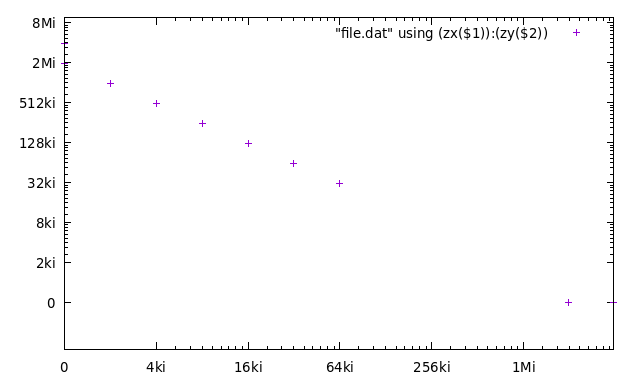When plotted properly, the zero value would be at $\log(0) = -\infty$ on the respective axis, which is not very practical on finite screens. On the other hand, logarithmic scale is a good way how to represent values spanning multiple orders of magnitude and there are situations where the zero value is valid.

This also shows how to use binary prefix format (such as Ki, Mi,…).TODO Learn how to implement this with set nonlinear x via f(x) inverse F(x).